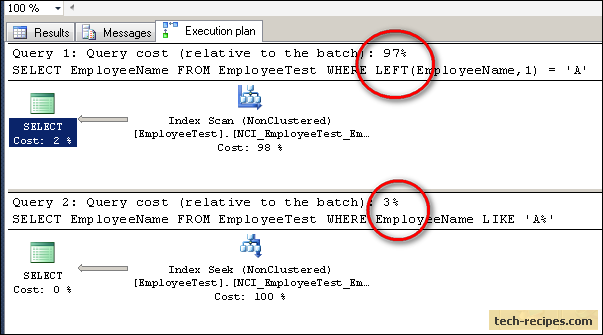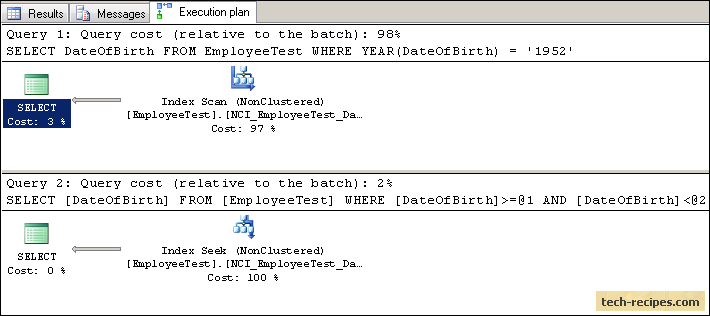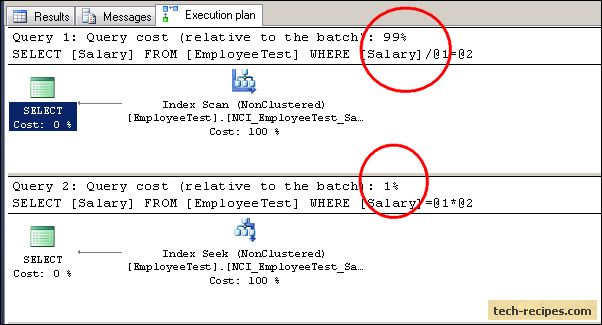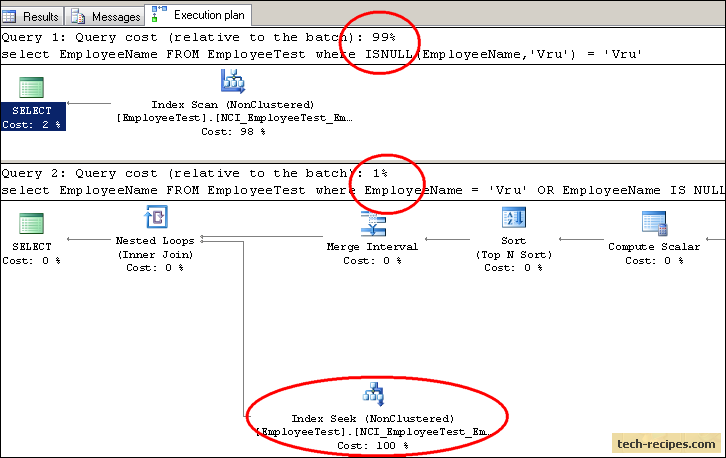# Sargable Queries in SQL Server with ExamplesThe most interesting part of my job is performance tuning and optimization in T-SQL. The heart of performance tuning in an SQL server is proper and usable indexing on tables through the use of Sargable queries.

Sometimes, the Senior Database Developer at work tells me just to add an index to an xyz column because it is being used in multiple Where clauses within multiple SQL queries. That is when I need to calm my mind. If adding an index on the xyz column could solve every performance issue, then thousands of books would not have been published on T-SQL performance tuning, and I would have gone hunting for anaconda in the Amazon rainforest.

Sargable Queries (Search Argumentable)

In simple terms, Sargable queries are those which are able to use created indexes on them for faster searches and execution of a query.
A faster search means making an effective index seek out large numbers of rows and avoiding costly index scans.

Index Seek – queries are able to use indexes effectively and locate rows with less effort from the query optimizer.
Index Scan – scanning the entire table to locate rows to satisfy the search criteria

What Makes a Query Non-Sargable (not able to use created indexes effectively)?

1. using functions in Where clause conditions (because a function is evaluated against each row which forces the query optimizer not to use the index)
2. using LIKE ‘%Proposal%’ in Wild card search queries
3. performing arithmetic calculation on an index column in a Where clause

Let’s create and populate a table with 0.1 Million rows to see how to make queries Sargable.

This script would take time to create sample data based on your hardware configuration (3-5 minutes).

```-- Create a table with primary key
CREATE TABLE EmployeeTest (
id INT IDENTITY(1 ,1) PRIMARY KEY,
Salary INT,
DateOfBirth DATETIME,
EmployeeName VARCHAR( 80)
);
GO

-- Insert rows with random values
DECLARE @row INT ;
DECLARE @string VARCHAR (80), @length INT, @code INT;
SET @row = 0;
WHILE @row < 100000 BEGIN
SET @row = @row + 1;

IF @row = 10000
PRINT 'Rows inserted: '+CONVERT (VARCHAR( 20),@row );
IF @row = 20000
PRINT 'Rows inserted: '+CONVERT (VARCHAR( 20),@row );
IF @row = 30000
PRINT 'Rows inserted: '+CONVERT (VARCHAR( 20),@row );
IF @row = 40000
PRINT 'Rows inserted: '+CONVERT (VARCHAR( 20),@row );
IF @row = 50000
PRINT 'Rows inserted: '+CONVERT (VARCHAR( 20),@row );
IF @row = 60000
PRINT 'Rows inserted: '+CONVERT (VARCHAR( 20),@row );
IF @row = 70000
PRINT 'Rows inserted: '+CONVERT (VARCHAR( 20),@row );
IF @row = 80000
PRINT 'Rows inserted: '+CONVERT (VARCHAR( 20),@row );
IF @row = 90000
PRINT 'Rows inserted: '+CONVERT (VARCHAR( 20),@row );
IF @row = 100000
PRINT 'Done, Rows inserted: '+CONVERT (VARCHAR( 20),@row );

-- Build the random string
SET @length = ROUND (80* RAND(),0 );
SET @string = '' ;
WHILE @length > 0 BEGIN
SET @length = @length - 1 ;
SET @code = ROUND( 32*RAND (),0) - 6 ;
IF @code BETWEEN 1 AND 26
SET @string = @string + CHAR(ASCII ('a')+ @code-1 );
ELSE
SET @string = @string + ' ';
END

SET NOCOUNT ON ;
INSERT INTO EmployeeTest VALUES (
ROUND(2000000 *RAND()+ 10000,0 ),
CONVERT(DATETIME , ROUND (60000* RAND()-30000 ,9)),
@string
)

END
GO
```

Let’s create a Non-clustered index on every column.

```CREATE NONCLUSTERED INDEX [NCI_EmployeeTest_Salary] ON [dbo]. [EmployeeTest]
(
[Salary] ASC
)
GO

CREATE NONCLUSTERED INDEX [NCI_EmployeeTest_DateOfBirth] ON [dbo]. [EmployeeTest]
(
[DateOfBirth] ASC
)
GO

CREATE NONCLUSTERED INDEX [NCI_EmployeeTest_EmployeeName] ON [dbo]. [EmployeeTest]
(
[EmployeeName] ASC
)
GO
```

Let’s see some samples queries to see the difference between sargable and non-sargable queries.

1. Filtering the Result Based On the Employee Names Which Start with A

```SET STATISTICS IO ON

--Non-Sargable Query because of Function Used in Where Clause
SELECT EmployeeName
FROM  EmployeeTest
WHERE LEFT( EmployeeName,1 ) = 'A';

--Sargable Query
SELECT EmployeeName
FROM  EmployeeTest
WHERE EmployeeName LIKE 'A%';

SET STATISTICS IO OFF
```

The statistics below show the first non-sargable query took 680 logical reads, whereas the sargable query with a wild card search did only 25 logical reads.

``````(3115 row(s) affected)

(3115 row(s) affected)

The execution plans below show the first Non-Sargable query takes 97% cost, whereas the Sargable query takes 3% with Index Seek.2. Filtering Results for Specific a Year

```SET STATISTICS IO ON

--Non-Sargable Query because of Function Used in Where Clause
SELECT DateOfBirth
FROM  EmployeeTest
WHERE YEAR (DateOfBirth) = '1952';

--Sargable Query
SELECT DateOfBirth
FROM  EmployeeTest
WHERE DateOfBirth >= '19520101' AND DateOfBirth < '19530101';

SET STATISTICS IO OFF
```

The statistics below show the first non-Sargable query took 226 logical reads, whereas the Sargable query completed only four logical reads.

``````(628 row(s) affected)

(628 row(s) affected)

The Execution Plans below show the first Non-Sargable query takes 98% Batch cost with Index Scan, whereas the Sargable query takes 2% with Index Seek.3. Calculations on an Index Column in a Where Clause

```SET STATISTICS IO ON

--Non-Sargable Query because of Calculation done on Index Column
--in Where Clause

SELECT Salary
FROM  EmployeeTest
WHERE Salary / 2 = 50147 ;

--Sargable Query

SELECT Salary
FROM  EmployeeTest
WHERE Salary  = (50147 * 2);

SET STATISTICS IO OFF
```

The statistics below show the first non-sargable query took 178 logical reads, whereas the sargable query completed only two logical reads.

``````(3 row(s) affected)

(3 row(s) affected)

The execution plans below show the first Non-sargable query takes 99% Batch cost with Index Scan, whereas the Sargable query takes 1% with Index Seek.4. Using the ISNULL Function in a Where Clause

```SET STATISTICS IO ON

--Non-Sargable Query because of ISNULL function on Index Column
--in Where Clause

select EmployeeName
FROM   EmployeeTest
where  ISNULL (EmployeeName, 'Vru') = 'Vru' ;

--Sargable Query

select EmployeeName
FROM   EmployeeTest
where  EmployeeName = 'Vru' OR EmployeeName IS NULL;

SET STATISTICS IO OFF
```

The statistics below show the first non-sargable query took 680 logical reads, whereas the sargable query completed only six logical reads.

``````(1 row(s) affected)

(1 row(s) affected)

The execution plans below show the first Non-sargable query takes 99% Batch cost with Index Scan, whereas the Sargable query takes 1% with Index Seek.